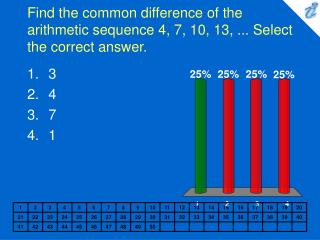# Find the common difference of the arithmetic sequence 4, 7, 10, 13, ... Select the correct answer. - PowerPoint PPT PresentationDownload PresentationFind the common difference of the arithmetic sequence 4, 7, 10, 13, ... Select the correct answer.

Find the common difference of the arithmetic sequence 4, 7, 10, 13, ... Select the correct answer.
Download Presentation## Find the common difference of the arithmetic sequence 4, 7, 10, 13, ... Select the correct answer.

- - - - - - - - - - - - - - - - - - - - - - - - - - - E N D - - - - - - - - - - - - - - - - - - - - - - - - - - -
##### Presentation Transcript

1. Find the common difference of the arithmetic sequence 4, 7, 10, 13, ... Select the correct answer. • 3 • 4 • 7 • 1

2. Find the n-th term of the arithmetic sequence. 4, 4 + s, 4 + 2s, 4 + 3s, ... Select the correct answer. • 4 + s(n - 1) • 4 + sn • s + 4(n - 1) • s + 4n

3. The 50th term of an arithmetic sequence is 67, and the common difference is 3. Find the first three terms. Select the correct answer. • -80, -77, -74 • -80, -74, -68 • -83, -77, -71 • -83, -80, -77

4. A partial sum of an arithmetic sequence is given. Find the sum. 5 + 8 + 11 + ... + 62. Choose the answer from the following: • 670 • 1,340 • 700 • 86

5. A man gets a job with a salary of \$30,000 a year. He is promised a \$2,100 raise each subsequent year. Find his total earnings for a 15 year period. Choose the answer from the following: • \$670,500 • \$1,341,000 • \$686,250 • \$61,500